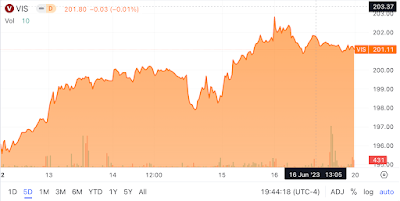Showing posts with label Investments. Show all posts
Showing posts with label Investments. Show all posts

## Thursday, December 15, 2022

### J.M. Smucker's Low Correlation With The Vanguard S&P 500 Index ETF

J.M. Smucker is known for its iconic and timeless consumer staples brands (Exhibit 1)

Note: Click on each image in this blog post to view an enlarged version

Exhibit 1: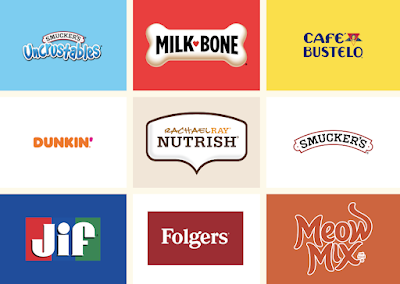Brands Owned by J.M. Smucker & Co. (Source: J.M. Smucker)

Here's the histogram of monthly returns for J.M. Smucker between June 2019 and November 2022 (Exhibit 2).

Exhibit 2: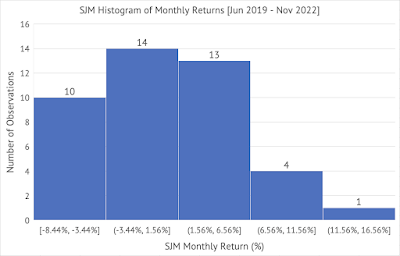J.M. Smucker (SJM) Histogram of Monthly Returns (Source: Data provided by IEX Cloud, author calculations & graph using Microsoft Excel)

The average monthly return for J.M. Smucker is less than the Vanguard S&P 500 Index ETF (Exhibit 3).
Exhibit 3: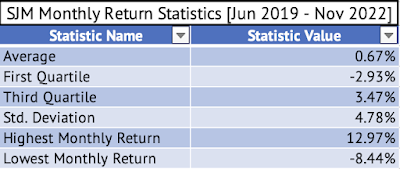J.M. Smucker Monthly Return Statistics - Average, First Quartile, Third Quartile, Standard Deviation, Highest Monthly Return, Lowest Monthly Return (Source: Data provided by IEX Cloud, author calculations & graph using Microsoft Excel)

J.M. Smucker had a lower standard deviation than the Vanguard ETF during this period (Exhibit 4). A company with a lower standard deviation than the well-diversified ETF, a measure of volatility, is an infrequent occurrence.

Exhibit 4: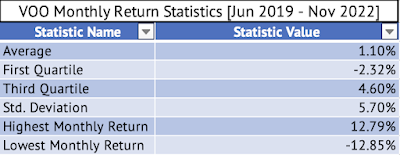Vanguard S&P 500 Index ETF Monthly Return Statistics - Average, First Quartile, Third Quartile, Standard Deviation, Highest Monthly Return, Lowest Monthly Return (Source: Data provided by IEX Cloud, author calculations & graph using Microsoft Excel)

Here's a graph of the monthly returns of the Vanguard ETF (x-axis) and J.M.Smucker (y-axis) with the fitted regression line (Exhibit 5).
Exhibit 5: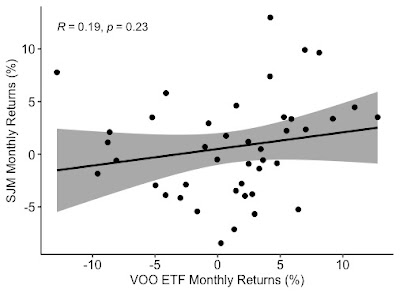Monthly Return Graph of the Vanguard S&P 500 Index ETF and J.M. Smucker (Source: Data provided by IEX Cloud, author calculations & graph using Microsoft Excel & RStudio)

The correlation of the monthly returns between June 2019 and November 2022 between the Vanguard ETF and J.M. Smucker is a low 0.19 (Exhibit 5). The fitted linear regression line has a p-value of 0.23, indicating that the relationship is insignificant at the 95% confidence interval.

The fitted linear regression line has a p-value of 0.23, indicating that the relationship is insignificant at the 95% confidence interval. Here's the output from the linear regression:

> summary(lmVOOSJM)

Call:
lm(formula = SJM_Monthly_Return ~ VOO_Monthly_Return, data = VOOandSJM)

Residuals:
Min        1Q    Median        3Q       Max
-0.089842 -0.034389  0.002403  0.022171  0.118077

Coefficients:
Estimate  Std. Error t value  Pr(>|t|)
(Intercept)        0.005011   0.007476   0.670    0.507
VOO_Monthly_Return 0.157911   0.130216   1.213    0.232

Residual standard error: 0.04755 on 40 degrees of freedom
Multiple R-squared:  0.03546, Adjusted R-squared:  0.01135
F-statistic: 1.471 on 1 and 40 DF,  p-value: 0.2324

The beta for J.M. Smucker is 0.15, but the high p-value is a concern. This beta (the coefficient of VOO_Monthly_Return) may not be the true value. Yahoo Finance has calculated a beta of 0.24.

## Saturday, December 10, 2022

### Monthly Return Analysis of Conagra Brands

Conagra Brands owns many iconic brands in the food business (Exhibit 1). The company is categorized as a consumer staple.

Exhibit 1:

Here's the histogram of monthly returns of Conagra Brands between June 2019 and November 2022 (Exhibit 2). Please click on the image to see an enlarged version.

Exhibit 2: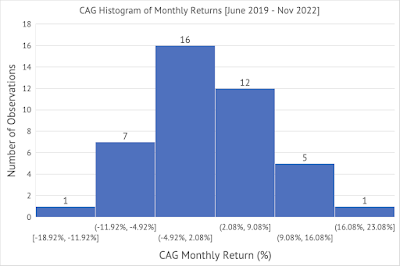Conagra Brands Histogram of Monthly Returns (Source: Data Provided by IEX Cloud, Author Calculations using Excel)The average monthly returns of Conagra Brands (Exhibit 3) are very similar to that of the Vanguard S&P 500 Index ETF (Exhibit 4).Exhibit 3: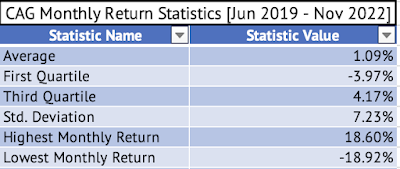(Source: Data Provided by IEX Cloud, Data Calculations Using Excel)Exhibit 4: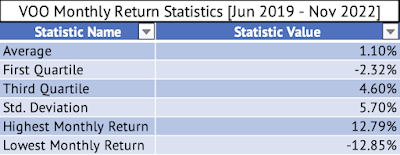(Source: Data Provided by IEX Cloud, Data Calculations Using Excel)The monthly returns of Conagra Brands and the Vanguard S&P 500 Index ETF have a mild positive correlation of 0.27 (Exhibit 5). Exhibit 5:A 12-month rolling correlation of the monthly returns yielded a very high positive correlation of 0.8 between April 2020 and March 2021 (Exhibit 6).Exhibit 6: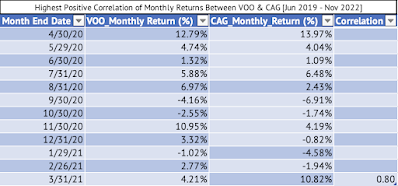(Source: Data Provided by IEX Cloud, Correlation Calculations Using RStudio)A 12-month rolling correlation of the monthly returns yielded the highest negative correlation of 0.37 between July 2021 and June 2022 (Exhibit 7).Exhibit 7: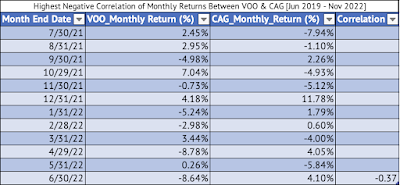(Source: Data Provided by IEX Cloud, Correlation Calculations Using RStudio)A linear regression model estimates Conagra's Beta at 0.34, which is not statistically significant at the 95% confidence interval. The p-value is 0.083, suggesting that the correlation is not statistically significant.Here's the output of the linear model:Call:lm(formula = CAG_Monthly_Return ~ VOO_Monthly_Return, data = VOOandCAG)Residuals:      Min        1Q    Median        3Q       Max -0.168638  -0.044057  -0.004737   0.045175  0.170379 Coefficients:                   Estimate Std. Error t value Pr(>|t|)  (Intercept)        0.007141   0.011079   0.645   0.5229  VOO_Monthly_Return 0.342593   0.192981   1.775   0.0835 .---Signif. codes:  0 ‘***’ 0.001 ‘**’ 0.01 ‘*’ 0.05 ‘.’ 0.1 ‘ ’ 1Residual standard error: 0.07047 on 40 degrees of freedomMultiple R-squared:  0.07303, Adjusted R-squared:  0.04986 F-statistic: 3.152 on 1 and 40 DF,  p-value: 0.08346The adjusted R-squared is 0.049, meaning that just 4.9% of Conagra's monthly returns can be explained by the monthly returns of the Vanguard S&P 500 Index ETF.

## Sunday, December 4, 2022

### Mothly Return Volatility (Beta) of Reynolds Consumer Products

Reynolds Consumer Products (REYN) makes many iconic household products, such as Reynolds Wrap, Hefty waste bags, and FreshLock zipper bags [Exhibit 1]

Exhibit 1: Some of the Products Made by Reynolds Consumer Products Co.Reynolds Consumer Products Source: Reynolds brands

I analyzed the monthly return of Reynolds (REYN) between February 2020 and November 2022. Here's the histogram of the monthly returns (click on the image to see an enlarged version) [Exhibit 2]:

Exhibit 2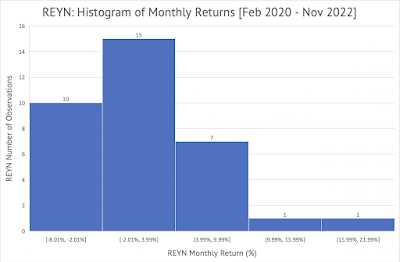Source: Data Provided by IEX Cloud, Author Calculations and Graphs Using Microsoft Excel

Here's the graph of the monthly returns of the Vanguard S&P 500 Index ETF (VOO) on the x-axis and Reynold's monthly returns on the y-axis [Exhibit 3]:

Exhibit 3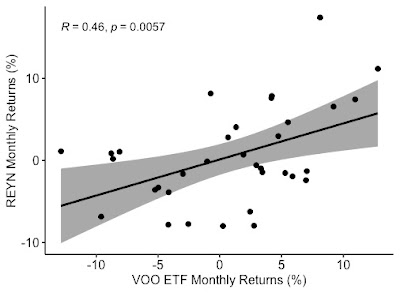Source: Data Provided by IEX Cloud, Graph Created using RStudio

The Pearson correlation of the monthly returns is a positive 0.46. This correlation value can be considered to have medium strength. This correlation is statistically significant at the 95% confidence interval with a p-value of 0.0057.

A linear regression of the monthly returns of Reynolds and the Vanguard S&P 500 Index ETF yields a beta value of 0.44. This beta value means that for every 1% change in the value of the Vanguard ETF, on average, Reynolds' stock will change by 0.44%. Yahoo Finance also shows a beta of 0.44 [Exhibit 4]

Exhibit 4

Source: Yahoo Finance

The adjusted R-squared value provided by the linear regression is 0.19. This adjusted R-squared value indicates that about 19% of Reynold's monthly returns are explained by the monthly returns of the Vanguard S&P 500 Index ETF.

Here's the output from the linear regression model constructed using RStudio:

Call:

lm(formula = REYN_Monthly_Return ~ VOO_Monthly_Return, data = VOOandREYN_MonthlyReturns)

Residuals:

Min        1Q    Median        3Q       Max

-0.092919 -0.037524 -0.003499  0.037494  0.137349

Coefficients:

Estimate Std. Error t value Pr(>|t|)

(Intercept)        0.001072   0.009234   0.116  0.90828

VOO_Monthly_Return 0.440311   0.148653   2.962  0.00572 **

---

Signif. codes:  0 ‘***’ 0.001 ‘**’ 0.01 ‘*’ 0.05 ‘.’ 0.1 ‘ ’ 1

Residual standard error: 0.05329 on 32 degrees of freedom

Multiple R-squared:  0.2152, Adjusted R-squared:  0.1907

F-statistic: 8.773 on 1 and 32 DF,  p-value: 0.005722

The p-value is significant at a 95% confidence interval with a value of 0.005722.

Here's the residuals plot for the linear regression between Reynolds Consumer Products and the Vanguard S&P 500 Index ETF [Exhibit 5]:

Exhibit 5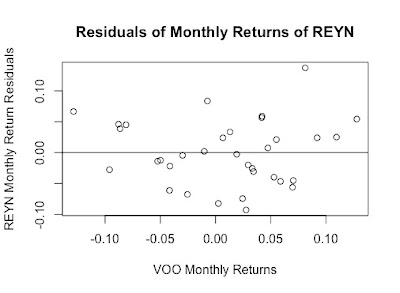Residuals Plot for Linear Regression of the monthly returns of the Vanguard S&P 500 Index as the independent variable and Reynold Consumer Products (Source: Data Provided by IEX Cloud, Graph Created using RStudio)

Here are the average, first quartile, third quartile, and standard deviation of Reynold's monthly returns [Exhibit 6]:

Exhibit 6

Here are the average, first quartile, third quartile, and standard deviation of the Vanguard S&P 500 Index ETF [Exhibit 7]:

## Saturday, February 26, 2022

### Welch Two Sample t-test of Daily Price Returns of J.M. Smucker and Mondelez

J.M. Smucker and Mondelez are consumer staples companies with similar financial performance. Both companies compete in similar product categories, especially when it relates to snacks. But, Mondelez has a vast international operation, while J. M. Smucker is more focused on the U.S. with very little So, I wanted to see if the mean daily returns are the same between the two stocks.

First, I downloaded the daily price return data for the past six months for both J.M. Smucker (SJM) and Mondelez (MDLZ) from IEX Cloud

I did a correlation between the daily price returns of the two companies using R:

> cor(SJM_6Month_ClosingPrice_Daily_Return_Data\$changePercent, MDLZ_6Month_ClosingPrice_Daily_Return_Data\$changePercent)

 0.5815403

The correlation in the daily returns between the two companies was 0.58 for the past six months. It is a positive correlation, but I would not consider this as a strong correlation in returns between the two companies. I would consider it a strong correlation if it was at or above 0.70. This came as a surprise given that both the companies are in the consumer staples sector and that sector has performed very well since December 2021.

Next, I wanted to calculate the p-value for the Welch two-sample t-test between means of the daily returns.  The null hypothesis is that the difference in mean daily returns between J.M. Smucker and Mondelez is zero. The alternate hypothesis is that the difference is not zero. The p-value is 0.9035, which means the null hypothesis can be resoundingly rejected. The t-test command in R and the result set is presented below. The J. M. Smucker had a mean daily return of 0.0893% and Mondelez had a daily return of 0.0717%. There is a 1.7 basis points difference in returns with J. M. Smucker having a slightly larger daily return than Mondelez.

> t.test(SJM_6Month_ClosingPrice_Daily_Return_Data\$changePercent,         MDLZ_6Month_ClosingPrice_Daily_Return_Data\$changePercent)

Welch Two Sample t-test

data:  SJM_6Month_ClosingPrice_Daily_Return_Data\$changePercent and MDLZ_6Month_ClosingPrice_Daily_Return_Data\$changePercent

t = 0.1214, df = 239.4, p-value = 0.9035

alternative hypothesis: true difference in means is not equal to 0

95 percent confidence interval:

-0.002682718  0.003035099

sample estimates:

mean of x    mean of y

0.0008936508 0.0007174603

Finally, here's the scatter plot of daily price change of J.M. Smucker (SJM) and Mondelez (MDLZ).

(Data Source: IEXCloud.io, Plot Created using RStudio)

### The Industrials Sector is on a Tear

The Vanguard Industrials Index ETF ( VIS ) touched a 52-week high of \$202.86 on Friday, June 16 (Exhibit 1) .   Exhibit 1: Vanguard Industr...